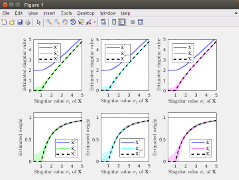[english]
[français]

# Generalized SURE for optimal shrinkage of singular values in low-rank matrix denoising

### Description• We consider the problem of estimating a low-rank signal matrix from noisy measurements under the assumption that the distribution of the data matrix belongs to an exponential family. In this setting, we derive generalized Stein's unbiased risk estimation (SURE) formulas that hold for any spectral estimators which shrink or threshold the singular values of the data matrix. This leads to new data-driven spectral estimators, whose optimality is discussed using tools from random matrix theory and through numerical experiments. Under the spiked population model and in the asymptotic setting where the dimensions of the data matrix are let going to infinity, some theoretical properties of our approach are compared to recent results on asymptotically optimal shrinking rules for Gaussian noise. It also leads to new procedures for singular values shrinkage in finite-dimensional matrix denoising for Gamma-distributed and Poisson-distributed measurements.

### Associated publications and source codes

Associated publications/reports:
• Jérémie Bigot, Charles Deledalle & Delphine Féral.
Generalized SURE for optimal shrinkage of singular values in low-rank matrix denoising
Journal of Machine Learning Research, vol. 18, no. 137, pp. 1-50, 2017 (JMLR, ArXiv)
Presented at ISNPS'2018 (slides)

Associated Matlab's script:
up

Last modified: Tue Jan 29 15:58:03 Europe/Paris 2019